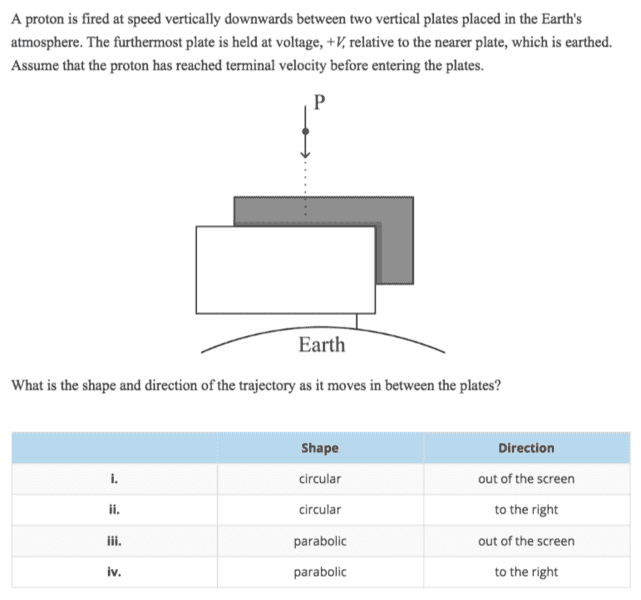# Trajectory of a proton as it moves between the plates

## Homework Statement## Homework Equations

What makes the shape circular/ parabolic?
what determines the direction?

## The Attempt at a Solution

Because the furthermost plate is positive, the proton would be repelled towards the screen, so i or iii. How do I know the shape?

#### Attachments

kuruman
Homework Helper
Gold Member
How do I know the shape?
What kind of acceleration generates circular motion? What about parabolic motion? Which do you have here?

What kind of acceleration generates circular motion? What about parabolic motion? Which do you have here?
Hi, is it because the proton is accelerating along the field lines not perpendicular to them? so it is a parabolic motion?

kuruman
Homework Helper
Gold Member
You provided a valid argument for parabolic motion. To answer with certainty, you need to provide an argument for excluding circular motion. What is that argument?

You provided a valid argument for parabolic motion. To answer with certainty, you need to provide an argument for excluding circular motion. What is that argument?
ummmm.... I'm not sure. Has it got something to do with the proton reaching terminal velocity before the plates?

kuruman
Homework Helper
Gold Member
ummmm.... I'm not sure. Has it got something to do with the proton reaching terminal velocity before the plates?
Nope. That's there to indicate that the vertical component of the velocity is constant. What kind of acceleration does an object in a circular path have? What's the difference between that kind of acceleration and the kind you have here?

Nope. That's there to indicate that the vertical component of the velocity is constant. What kind of acceleration does an object in a circular path have? What's the difference between that kind of acceleration and the kind you have here?
Centripetal acceleration? And this one is just uniform?

kuruman
Homework Helper
Gold Member
Correct on both accounts. Centripetal acceleration changes direction to stay perpendicular to the velocity. Here the direction of the acceleration is fixed in space and is perpendicualr to the velocity instantaneously only when the proton enters the plate region.

Correct on both accounts. Centripetal acceleration changes direction to stay perpendicular to the velocity. Here the direction of the acceleration is fixed in space and is perpendicualr to the velocity instantaneously only when the proton enters the plate region.
I am a bit confused - isn't the acceleration zero here? (because it is at terminal velocity?)

kuruman
Homework Helper
Gold Member
How many different forces act on the proton?

How many different forces act on the proton?
gravity and repulsion from the positive plate?

kuruman